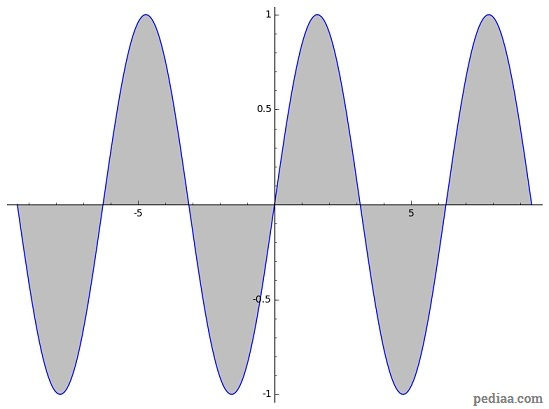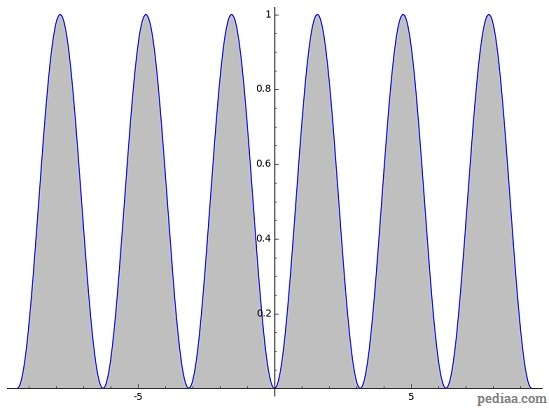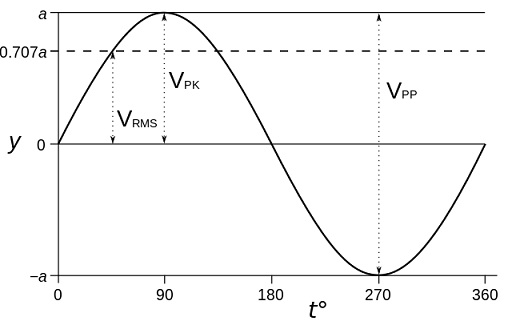# Difference Between RMS and Peak

## Main Difference – RMS vs. Peak

In alternating currents, the magnitude of current is always changing. Therefore, the current can be described by not just one, but several numbers. RMS and peak are two numbers that can be used to express an alternating current. The main difference between RMS and Peak is that peak refers to the maximum value that the current can reach in an alternating current whereas RMS is the peak current divided by the square root of two.

## What is Peak

Alternating currents change sinusoidally with time. Peak refers to the maximum value that the sinusoidally-varying current or voltage reaches. If the voltage$V(t)$ is expressed in the form$V(t)=V_0\mathrm {sin}\left( \omega t\right)$, then the peak voltage is$V_0$.

Peak-to-peak refers to the absolute value of the difference between maximum and minimum voltages. Peak-to-peak voltages are sometimes used to describe alternating currents. If the wave is sinusoidal, then$V_{peak-to-peak}=2\times V_{peak}$.

## What is RMS

RMS stands for Root Mean Square. Root mean squares are used to express averages of a quantity when the quantity can take negative and positive values. This is necessary so that negative values of a quantity do not cancel any positive quantities. Root mean squares are used in thermodynamics; for examples, to express the average velocity of gas molecules.

Since the voltage in an AC current varies sinusoidally, if we are to take the average voltage, we would get an answer of zero:The area under a sin curve averages out to be 0.

Instead, we square the current. Now, the average of the squared current is not 0, but a half:A sin squared curve. This has an average at 1/2.

Suppose a voltage is expressed as$V=V_{peak}\mathrm{sin}\left( \omega t\right)$

Suppose we want to find the average value of$V$. As we discussed earlier, one approach would be to first square the voltage. We do this now to both sides of the equation:$V^2={V_{peak}}^2\mathrm{sin}^2\left( \omega t\right)$

Next, we take the averges from both sides of the equation. The average of$\mathrm{sin}^2\left( \omega t\right)$ is$\frac{1}{2}$. So,${V^2}_{mean}={{V_{peak}}^2}\times \frac{1}{2}$

If we want to find the mean voltage, then we take the square roots:${V}_{mean}=\frac{V_{peak}}{\sqrt {2}}$

The figure below illustrates the peak, peak-to-peak and RMS voltages in an alternating current.A diagram showing peak, peak-to-peak and RMS voltages for a sine wave (PK=peak, PP=peak-to-peak, RMS=root mean square).

RMS voltage is useful in calculating the average power in a circuit. The average power$P_{av}$ is given by$P_{av}=\frac{{V_{rms}}^2}{R}$. In terms of the RMS current, the average power is given by$P_{av}={I_{rms}}^2R$.

## Difference Between RMS and Peak

DPeak refers to the maximum value that the current or voltage reaches in an alternating current. RMS gives an average value for current or voltage.

When a voltage value for an AC current is quoted, it is usually the RMS value that is quoted.

RMS values are always smaller than peak values.

Image Courtesy:

“Graph of a sine wave voltage versus time (in degrees of angle) with RMS, peak, and peak-to-peak marked.” by AlanM1
(Derived from File:Sine wave 2.svg by en:User:Booyabazooka (CC0-licensed)) [CC0 1.0], via Wikimedia Commons